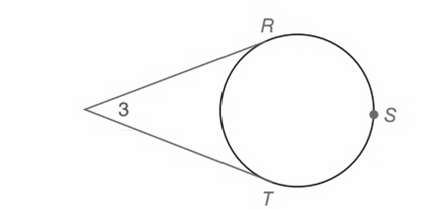Chapter 6.CT, Problem 6CTElementary Geometry For College St...

7th Edition
Alexander + 2 others
ISBN: 9781337614085

Solutions

Chapter
SectionElementary Geometry For College St...

7th Edition
Alexander + 2 others
ISBN: 9781337614085
Textbook Problem

Given the tangents with m R T ⌢ = 146 ° , find :a) m R S T ⌢ b) m ∠ 3To determine

(a)

To find:

mRST.

Explanation

Approach:

The degree measured around a circle is 360°.

Calculation:

Given:

mRT=146°

Consider the given figure,

To determine

(b)

To find:

m3.

Still sussing out bartleby?

Check out a sample textbook solution.

See a sample solution

The Solution to Your Study Problems

Bartleby provides explanations to thousands of textbook problems written by our experts, many with advanced degrees!

Get Started

In Exercises 4144, determine whether the statement is true or false. 44. 561112

Applied Calculus for the Managerial, Life, and Social Sciences: A Brief Approach

4. (a) Write in exponential form and find x. (b) Write in logarithmic form and approximate x.

Mathematical Applications for the Management, Life, and Social Sciences

Use the guidelines of this section to sketch the curve. y=(x1)2x2+1

Single Variable Calculus: Early Transcendentals, Volume I

Solve for x: ln(e + x) = 1. a) 0 b) 1 c) e d) ee e

Study Guide for Stewart's Single Variable Calculus: Early Transcendentals, 8th